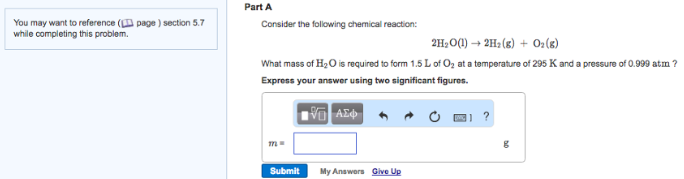# Problem: Part AConsider the following chemical reaction: 2H2O(l) → 2H2(g) + O2(g) What mass of H2O is required to form 1.5 L of O2 at a temperature of 295 K and a pressure of 0.999 atm?Express your answer using two significant figures.

###### FREE Expert Solution
86% (325 ratings)###### Problem Details

Part A

Consider the following chemical reaction:

2H2O(l) → 2H2(g) + O2(g)

What mass of H2O is required to form 1.5 L of O2 at a temperature of 295 K and a pressure of 0.999 atm?